# Apply State-Space Methodology to Analyze Diebold-Li Yield Curve Model

This example shows how to use state-space models (SSM) and the Kalman filter to analyze the Diebold-Li yields-only and yields-macro models  of monthly yield-curve time series derived from U.S. Treasury bills and bonds. The analysis includes model estimation, simulation, smoothing, forecasting, and dynamic behavior characterization by applying Econometrics Toolbox™ SSM functionality. The example compares SSM estimation performance to the performance of more traditional econometric estimation techniques.

After the financial crisis of 2008, solvency regulations placed greater emphasis on market valuation and accounting of liabilities. As a result, many financial firms, especially insurance companies and pension funds, write annuity contracts and incur long-term liabilities that call for sophisticated approaches to model and forecast yield curves.

Because the value of long-term liabilities greatly increases with low interest rates, the probability of very low yields must be modeled accurately. The Kalman filter, with its ability to incorporate time-varying coefficients and infer unobserved factors driving the evolution of observed yields, is often appropriate for the estimating of yield curve model parameters and then simulating and forecasting yields, which are integral to insurance and pension analysis.

In this example, you build, fit, and analyze a yield curve model by using Econometrics Toolbox SSM functionality and this workflow:

1. Represent the Diebold-Li yields-only model in parametric state-space model form, as supported by Econometrics Toolbox SSM functionality.

2. For the yields-only model, reproduce the in-sample estimation results published in , and compare the results obtained to those of the two-step approach as published in .

3. For the yields-only model, compute minimum mean square error (MMSE) forecasts and show the Monte Carlo simulation capabilities of the SSM functionality.

4. Estimate the yields-macro Diebold-Li SSM, which integrates the financial and macroeconomic factors.

5. Compute impulse response functions (IRF) and forecast error variance decompositions (FEVD) in the state-space framework, which characterize the yields-to-macro and macro-to-yields links.

6. Estimate the Diebold-Li SSM augmented with exogenous macroeconomic variables.

### Diebold-Li Yield Curve Model

The Diebold-Li model is a variant of the Nelson-Siegel model , reparameterized from the original formulation to contain yields only. For observation date $t$ and time to maturity $\tau$, the Diebold-Li model of yield ${y}_{t}\left(\tau \right)$ is

${y}_{t}\left(\tau \right)={L}_{t}+{S}_{t}\left(\frac{1-{e}^{-\lambda \tau }}{\lambda \tau }\right)+{C}_{t}\left(\frac{1-{e}^{-\lambda \tau }}{\lambda \tau }-{e}^{-\lambda \tau }\right),$

where:

• ${L}_{t}$ is the long-term factor (level).

• ${S}_{t}$ is the short-term factor (slope).

• ${C}_{t}$ is the medium-term factor (curvature).

• $\lambda$ determines the maturity at which the loading on the curvature is maximized and governs the exponential decay rate of the model.

### State-Space Formulation of Diebold-Li Model

#### Econometrics Toolbox SSM Form

The ssm object of the Econometrics Toolbox enables you to specify a linear problem in state-space representation. To perform the following operations on the SSM, pass the you ssm object that represents it to the appropriate function.

For state vector ${x}_{t}$ and observation (response) vector ${y}_{t}$, the parametric form of the Econometrics Toolbox SSM has the following linear state-space representation:

$\begin{array}{l}{x}_{t}={A}_{t}{x}_{t-1}+{B}_{t}{u}_{t}\\ {y}_{t}={C}_{t}{x}_{t}+{D}_{t}{\epsilon }_{t},\end{array}$

where the vectors ${u}_{t}$ and ${ϵ}_{t}$ are uncorrelated, unit-variance, white noise processes. In the SSM, the first equation is the state equation and the second is the observation equation. The model parameters ${A}_{t}$, ${B}_{t}$, ${C}_{t}$, and ${D}_{t}$ are the state-transition, state-disturbance-loading, measurement-sensitivity, and observation-innovation coefficient matrices, respectively.

Although the SSM functions accommodate time-varying (dynamic) parameters, parameters whose values and dimensions can change with time, in the Diebold-Li model, the parameters are time invariant (static).

#### Diebold-Li Yields-Only SSM

The level, slope, and curvature factors of the Diebold-Li model follow a vector autoregressive process of first order (VAR(1)), which forms a state-space system. Diebold, Rudebusch, and Aruoba  compose the state vector with the level, slope, and curvature factors. The resulting state transition equation, which governs the dynamics of the state vector, is

$\left[\begin{array}{c}{L}_{t}-{\mu }_{L}\\ {S}_{t}-{\mu }_{S}\\ {C}_{t}-{\mu }_{C}\end{array}\right]=\left[\begin{array}{ccc}{a}_{11}& {a}_{12}& {a}_{13}\\ {a}_{21}& {a}_{22}& {a}_{23}\\ {a}_{31}& {a}_{32}& {a}_{33}\end{array}\right]\left[\begin{array}{c}{L}_{t-1}-{\mu }_{L}\\ {S}_{t-1}-{\mu }_{S}\\ {C}_{t-1}-{\mu }_{C}\end{array}\right]+\left[\begin{array}{c}{\eta }_{Lt}\\ {\eta }_{St}\\ {\eta }_{Ct}\end{array}\right],$

where ${\mu }_{\mathit{j}}$, $\mathit{j}\in \left\{\mathit{L},\mathit{S},\mathit{C}\right\}$, is the mean of factor $\mathit{j}$. The corresponding observation (measurement) equation is

$\left[\begin{array}{c}{y}_{{\tau }_{1}t}\\ {y}_{{\tau }_{2}t}\\ ⋮\\ {y}_{{\tau }_{N}t}\end{array}\right]=\left[\begin{array}{ccc}1& \frac{1-{e}^{-\lambda {\tau }_{1}}}{\lambda {\tau }_{1}}& \frac{1-{e}^{-\lambda {\tau }_{1}}}{\lambda {\tau }_{1}}-{e}^{-\lambda {\tau }_{1}}\\ 1& \phantom{\rule{0.2777777777777778em}{0ex}}\frac{1-{e}^{-\lambda {\tau }_{2}}}{\lambda {\tau }_{2}}& \frac{1-{e}^{-\lambda {\tau }_{2}}}{\lambda {\tau }_{2}}-{e}^{-\lambda {\tau }_{2}}\\ ⋮& ⋮& ⋮\\ 1& \frac{1-{e}^{-\lambda {\tau }_{N}}}{\lambda {\tau }_{N}}& \frac{1-{e}^{-\lambda {\tau }_{N}}}{\lambda {\tau }_{N}}-{e}^{-\lambda {\tau }_{N}}\end{array}\right]\left[\begin{array}{c}{L}_{t}\\ {S}_{t}\\ {C}_{t}\end{array}\right]+\left[\begin{array}{c}{w}_{{\tau }_{1}t}\\ {w}_{{\tau }_{2}t}\\ ⋮\\ {w}_{{\tau }_{N}t}\end{array}\right].$

The Diebold-Li model has the following matrix representation for the 3-D vector of mean-adjusted factors ${f}_{t}$ and observed yields ${y}_{t}$:

$\begin{array}{c}\left({f}_{t}-\mu \right)=A\left({f}_{t-1}-\mu \right)+{\eta }_{t}\\ {y}_{t}=\Lambda {f}_{t}+{w}_{t}.\end{array}$

The Diebold-Li model imposes the following assumptions on the state-equation factor disturbances ${\eta }_{t}$ and the observation-equation innovations (deviations of observed yields at various maturities) ${w}_{t}$:

• ${\eta }_{t}$ and ${w}_{t}$ are orthogonal, Gaussian, white noise processes, symbolically

$\left[\begin{array}{c}{\eta }_{t}\\ {w}_{t}\end{array}\right]\phantom{\rule{0.5em}{0ex}}\sim WN\left(\left[\begin{array}{c}0\\ 0\end{array}\right],\left[\begin{array}{cc}Q& 0\\ 0& H\end{array}\right]\right).$

• Disturbances ${\eta }_{t}$ are contemporaneously correlated, which implies that their covariance matrix $Q$ is nondiagonal.

• Innovations in ${w}_{t}$ are uncorrelated, which implies the covariance matrix $H$ is diagonal.

Define the latent states ${\mathit{x}}_{\mathit{t}}$ as the mean-adjusted factors

${x}_{t}={f}_{t}-\mu ,$

and define the intercept-adjusted (deflated) yields $\stackrel{\sim }{\text{\hspace{0.17em}}{\mathit{y}}_{\mathit{t}}}$ as

${\underset{}{\overset{\sim }{y}}}_{t}={y}_{t}-\Lambda \mu .$

Substitute ${\mathit{x}}_{\mathit{t}}$ and $\stackrel{\sim }{\text{\hspace{0.17em}}{\mathit{y}}_{\mathit{t}}}$ into the preceding equations, and the resulting Diebold-Li state-space system is

$\begin{array}{l}{x}_{t}=A{x}_{t-1}+{\eta }_{t}\\ {\underset{}{\overset{\sim }{y}}}_{t}=\Lambda {x}_{t}+{w}_{t}.\end{array}$

Compare the Diebold-Li state-space system to the formulation that the Econometrics Toolbox SSM functionality supports, which is

$\begin{array}{l}{x}_{t}={A}_{t}{x}_{t-1}+{B}_{t}{u}_{t}\\ {y}_{t}={C}_{t}{x}_{t}+{D}_{t}{\epsilon }_{t}.\end{array}$

The state-transition coefficient matrix $A$ is the same in both formulations, and Diebold-Li model matrix $\Lambda$ is the same as the measurement-sensitivity coefficient matrix $C$ in the SSM formulation.

The relationship between the disturbance and innovation processes is more subtle. Because ${\eta }_{\mathit{t}}=\mathit{B}{\mathit{u}}_{\mathit{t}}$ and ${\mathit{w}}_{\mathit{t}}=\mathit{D}{\epsilon }_{\mathit{t}}$, the covariances of the random variables must be equal. As a result of the linear transformation property of Gaussian random vectors, the relationship between the covariances of the disturbances and innovations is

$\begin{array}{l}Q=B{B}^{\prime }\\ H=D{D}^{\prime }.\end{array}$

To prepare the Diebold-Li model for Econometrics Toolbox SSM functionality, use the ssm function to specify the SSM; ssm returns an ssm model object representing the model. The ssm function enables you to create a model containing known or unknown parameters; an ssm object containing unknown parameters is a template for estimation. You can either explicitly set the parameters, or you can specify a custom function that implicitly defines the SSM.

To create an SSM explicitly, you must specify all coefficient matrices $\mathit{A}$, $\mathit{B}$, $\mathit{C}$, and $\mathit{D}$. To indicate the presence and placement of unknown parameter values, specify NaN values. Each NaN entry corresponds to a unique parameter to estimate. This approach to model creation is convenient when each parameter affects and is uniquely associated with a single element of a coefficient matrix.

To create an SSM implicitly, you must specify a custom parameter-to-matrix mapping function that maps an input parameter vector to model parameters $\mathit{A}$, $\mathit{B}$, $\mathit{C}$, and $\mathit{D}$. The function content defines the model formulation. This approach to model creation is useful for complex models or for imposing parameter constraints.

An ssm object does not store nonzero offsets of state variables, or any parameters associated with regression components, in the observation equation. To estimate coefficients of a regression component, you must deflate the observations ${y}_{t}$. Similarly, other ssm functions expect deflated, or preprocessed, observations to account for any offsets or a regression component in the observation equation.

Because the Diebold-Li model has the following characteristics that are impossible to specify explicitly, this example creates an ssm object implicitly.

• Each factor in the Diebold-Li model includes a nonzero offset (mean), which represents a regression component.

• The model imposes a symmetry constraint on the covariance matrix $Q=B{B}^{\prime }$ and a diagonality constraint of the covariance matrix $H=D{D}^{\prime }$.

• The model includes a decay rate parameter $\lambda$.

The preceding state-space formulation is not unique. For example, you can include the factor offsets as states in the state equation instead of observation deflators. The advantage of observation deflation is that the dimensionality of the state vector directly corresponds to the 3-D yields-only factor model of Diebold, Rudebusch, and Aruoba . The disadvantage is that the estimation is performed on the deflated yields, and therefore you must account for the adjustment by deflating, and then inflating, the yields when you pass the estimated model to other ssm functions.

The yield data consists of 29 years of monthly, unsmoothed Fama-Bliss U.S. Treasury zero-coupon yield measurements, as analyzed and discussed in  and . The time series in the data represents maturities of 3, 6, 9, 12, 15, 18, 21, 24, 30, 36, 48, 60, 72, 84, 96, 108, and 120 months. The yields are expressed in percent and recorded at the end of each month, beginning January 1972 and ending December 2000, for a total of 348 monthly curves of 17 maturities each. For example, the time stamp 31-Jan-1972 corresponds to the beginning of February 1972. You can access the entire unsmoothed Fama-Bliss yield curve data set, a subset of which is analyzed in  and , at https://www.sas.upenn.edu/~fdiebold/papers/paper49/FBFITTED.txt.

This example uses the entire Diebold-Li data set Data_DieboldLi.mat to reproduce the estimation results published in , and it compares the results of the two-step and SSM approaches. Alternatively, you can analyze the forecast accuracy of the models by partitioning the data into in-sample and out-of-sample periods, and then fitting the models to the former set and assessing forecast performance of the estimated models using the latter set. For more details on assessing forecast accuracy of Diebold-Li models, see Tables 4 through 6 in .

Load the Diebold-Li data set, and then extract the yield series.

load Data_DieboldLi maturities = maturities(:); % Cast to a column vector Yields = DataTimeTable{:,1:17}; % In-sample yield series for estimation dates = DataTimeTable.Time; % Date stamps

### Estimate Yields-Only Diebold-Li Model Using Two-Step Method

Diebold and Li  estimate the parameters of their yield curve model by using a two-step approach:

1. Fix $\lambda$, and then, for each monthly yield curve, estimate the level, slope, and curvature parameters. The result is a 3-D time series of estimates of the unobserved level, slope, and curvature factors.

2. Fit a first-order autoregressive model to the time series of factors derived in the first step.

By fixing $\lambda$, the estimation procedure is ordinary least squares (OLS). Otherwise, the estimation procedure is nonlinear least squares. The Nelson-Siegel framework sets $\lambda$ = 0.0609 , which implies that loading on the curvature (medium-term factor) is maximized at 30 months.

Because the yield curve is parameterized as a function of the factors, forecasting the yield curve is equivalent to forecasting the underlying factors, and then evaluating the Diebold-Li model as a function of the factor forecasts.

The first step equates the three factors (level, slope, and curvature) to the regression coefficients obtained by OLS, and it accumulates a 3-D time series of estimated factors by repeating the OLS fit for each observed yield curve.

For each month (row), perform the first step by using OLS to fit the following linear model to the yield curve series.

${\mathit{y}}_{\mathit{j}}=\mathit{L}+\frac{1-{\mathit{e}}^{\lambda {\tau }_{\mathit{j}}}}{\lambda {\tau }_{\mathit{j}}}\mathit{S}+\left(\frac{1-{\mathit{e}}^{\lambda {\tau }_{\mathit{j}}}}{\lambda {\tau }_{\mathit{j}}}-{\mathit{e}}^{\lambda {\tau }_{\mathit{j}}}\right)\mathit{C}$, $\mathit{j}=\left\{3,6,9,...,120\right\}$.

Store the regression coefficients and residuals of the linear model fit.

lambda0 = 0.0609; X = [ones(size(maturities)) (1-exp(-lambda0*maturities))./(lambda0*maturities) ... ((1-exp(-lambda0*maturities))./(lambda0*maturities)-exp(-lambda0*maturities))]; Beta = zeros(size(Yields,1),3); Residuals = zeros(size(Yields,1),numel(maturities)); for i = 1:size(Yields,1) EstMdlOLS = fitlm(X,Yields(i,:)','Intercept',false); Beta(i,:) = EstMdlOLS.Coefficients.Estimate'; Residuals(i,:) = EstMdlOLS.Residuals.Raw'; end

Beta contains the 3-D time series of estimated factors.

Fit a first-order autoregressive (AR) model to the time series of estimated factors. You can accomplish this task in two ways:

• Fit a univariate AR(1) model to each factor separately, as in .

• Fit a VAR(1) model to all 3 factors simultaneously, as in .

Econometrics Toolbox supports univariate and multivariate AR estimation.

Fit a VAR(1) model to the estimated factors. For consistency with the SSM formulation, which works with the mean-adjusted factors, include an additive constant to account for the mean of each factor.

MdlVAR = varm(3,1); EstMdlVAR = estimate(MdlVAR,Beta);

EstMdlVAR is a varm model object representing the estimated VAR(1) factor model.

### Yields-Only Diebold-Li SSM Estimation

Next, estimate the Diebold-Li model using the implicit approach, in which you create and specify a parameter-to-matrix mapping function.

The mapping function Example_DieboldLi.m maps a parameter vector to SSM parameter matrices, deflates the observations to account for the means of each factor, and imposes constraints on the covariance matrices. For more details, open Example_DieboldLi.m.

Create an SSM by passing, to ssm, the parameter-to-matrix mapping function Example_DieboldLi as an anonymous function with an input argument representing the parameter vector params. The additional input arguments of the mapping function specify the yield and maturity information statically, which initialize the model for estimation.

Mdl = ssm(@(params)Example_DieboldLi(params,Yields,maturities));

Mdl is an ssm model object representing the SSM expressed in Example_DieboldLi. The model is solely a template for estimation.

The maximum likelihood estimation (MLE) of an SSM via the Kalman filter is widely known to be sensitive to the initial parameter values. Therefore, this example uses the results of the two-step approach to initialize the estimation.

You must pass the initial values required by estimate as a column vector. Construct the initial-value vector by performing the following procedure:

1. Specify the initial value for the coefficient matrix $\mathit{A}$ by stacking the estimated 3-by-3 AR coefficient matrix of the VAR(1) model columnwise.

2. For the initial value of the coefficient matrix $\mathit{B}$, the 3-by-3 covariance matrix $\mathit{Q}$ is the VAR(1) model innovations covariance matrix and $Q=B{B}^{\prime }$. Therefore, the estimate of $B$ is the lower Cholesky factor of $Q$. To ensure that $Q$ is symmetric, positive definite, and allows for nonzero off-diagonal covariances, allocate the six elements associated with the lower Cholesky factor of $Q$. In other words, this specification assumes that the covariance matrix $Q$ is nondiagonal, but it reserves space for the below-diagonal elements of the lower Cholesky factor of the covariance matrix so that $Q=B{B}^{\prime }$. Arrange the initial value along and below the main diagonal by stacking the matrix columnwise.

3. Because the covariance matrix $H$ in the Diebold-Li formulation is diagonal and $H=D{D}^{\prime }$, the matrix $D$ of the SSM is diagonal. Specify the initial value of $\mathit{D}$ as the square root of the diagonal elements of the sample covariance matrix of the residuals of the VAR(1) model, one element for each of the 17 maturities in the input yield data. Stack the initial value columnwise.

4. The $C$ matrix is a fully parameterized function of the estimated decay rate parameter $\lambda$. The mapping function computes it directly using $\lambda$, so $\mathit{C}$ does not require an initial value. Set the initial value of $\lambda$ to the traditional value 0.0609; the last element of the initial parameter column vector corresponds to it.

5. For the elements of the initial parameter vector associated with the factor means, set the sample averages of the OLS regression coefficients in the first step of the two-step approach.

6. Stack all initial values in the order ${\mathit{A}}_{0}$, ${\mathit{B}}_{0}$, ${\mathit{D}}_{0}$, ${\mu }_{0}$, and $\lambda$.

A0 = EstMdlVAR.AR{1}; A0 = A0(:); Q0 = EstMdlVAR.Covariance; B0 = [sqrt(Q0(1,1)); 0; 0; sqrt(Q0(2,2)); 0; sqrt(Q0(3,3))]; H0 = cov(Residuals); D0 = sqrt(diag(H0)); mu0 = mean(Beta)'; param0 = [A0; B0; D0; mu0; lambda0];

To facilitate estimation, set optimization options. Estimate the model by passing the ssm model template Mdl, the yield data, the initial values, and the optimization options to estimate. Turn off estimation displays. Because the covariance matrix $H=D{D}^{\prime }$ is diagonal, specify the univariate treatment of a multivariate series to improve the estimation run-time performance. The Kalman filter processes the vector-valued observations one at a time.

options = optimoptions('fminunc','MaxFunEvals',25000,'algorithm','quasi-newton', ... 'TolFun',1e-8,'TolX',1e-8,'MaxIter',1000,'Display','off'); [EstMdlSSM,params] = estimate(Mdl,Yields,param0,'Display','off', ... 'options',options,'Univariate',true); lambda = params(end); % Estimated decay rate mu = params(end-3:end-1)'; % Estimated factor means

EstMdlSSM is an ssm model object representing the estimated Diebold-Li SSM. The estimation procedure applies the Kalman filter.

### Compare Estimation Results

#### Estimated Parameters

Comparing the results of the two-step estimation method and the SSM fit helps you to understand the following characteristics:

• How closely the results of the two approaches agree

• How suitable the two-step estimation results are as initial parameter values for SSM estimation

Visually compare the estimated state-transition matrix $A$ of the SSM with the AR(1) coefficient matrix obtained from the VAR model.

EstMdlSSM.A
ans = 3×3 0.9944 0.0286 -0.0221 -0.0290 0.9391 0.0396 0.0253 0.0229 0.8415 
EstMdlVAR.AR{1}
ans = 3×3 0.9901 0.0250 -0.0023 -0.0281 0.9426 0.0287 0.0518 0.0125 0.7881 

The estimated coefficients closely agree. The diagonal elements are nearly 1, which indicates persistent self-dynamics of each factor. The off-diagonal elements are nearly 0, indicating weak cross-factor dynamics.

Next examine the state-disturbance-loading matrix $B$. Visually compare the corresponding estimated innovations covariance matrix $Q=B{B}^{\prime }$ from both estimation methods.

EstMdlSSM.B
ans = 3×3 0.3076 0 0 -0.0453 0.6170 0 0.1421 0.0255 0.8824 
QSSM = EstMdlSSM.B*EstMdlSSM.B'
QSSM = 3×3 0.0946 -0.0139 0.0437 -0.0139 0.3827 0.0093 0.0437 0.0093 0.7995 
QVAR = EstMdlVAR.Covariance
QVAR = 3×3 0.1149 -0.0266 -0.0719 -0.0266 0.3943 0.0140 -0.0719 0.0140 1.2152 

The estimated covariance matrices relatively agree. The estimated variances increase as the state proceeds from level to slope to curvature along the main diagonal.

Now compare the factor means from the estimation methods.

mu % SSM factor means
mu = 1×3 8.0246 -1.4423 -0.4188 
mu0' % Two-step factor means
ans = 1×3 8.3454 -1.5724 0.2030 

The estimated means of level and slope factors agree, but the curvature factor means differ in magnitude and sign.

#### Inferred Factors

The unobserved level, slope, and curvature factors (latent states) of the Diebold-Li model are integral to forecasting the evolution of future yield curves. In this part of the example, you examine the states inferred from each estimation method.

In the two-step estimation method, the latent states are the regression coefficients estimated in the OLS step.

In the SSM method, the smooth function implements backward smoothing of the Kalman filter algorithm: for $\mathit{t}=1,...,\mathit{T}$, the smoothed states are

$E\left({x}_{t}|{y}_{T},...,{y}_{1}\right).$

The SSM framework accounts for offset adjustments to the observed yields during estimation, as specified in the parameter-to-matrix mapping function. Specifically, the mapping function deflates the original observations, and therefore the estimate function works with the offset-adjusted yields ${\underset{}{\overset{\sim }{y}}}_{t}={y}_{t}-\Lambda \mu$ instead of the original yields ${\mathit{y}}_{\mathit{t}}$. The estimated SSM EstMdlSSM does not store data, and therefore it is agnostic of any adjustments made to the original yields. Therefore, when you call other SSM functions, such as filter or smooth, you must properly account for any offsets or a regression component associated with predictors that you include in the observation equation.

Infer the latent factors, while properly accounting for offsets, by following this procedure:

1. Deflate ${\mathit{y}}_{\mathit{t}}$ by subtracting the intercept associated with the estimated offset $C\mu =\Lambda \mu$. This action compensates for the offset adjustment that occurred during estimation.

2. Pass the estimated SSM EstMdlSSM and the deflated yields $\stackrel{\sim }{\text{\hspace{0.17em}}\mathit{y}{\text{\hspace{0.17em}}}_{\mathit{t}}}$ to smooth. The resulting smoothed state estimates correspond to the deflated yields.

3. Adjust the deflated, smoothed state estimates by adding the estimated mean $\mu$ to the factors. This action results in estimates of the unadjusted latent factors.

intercept = EstMdlSSM.C*mu'; DeflatedYields = Yields - intercept'; DeflatedStates = smooth(EstMdlSSM,DeflatedYields); EstimatedStates = DeflatedStates + mu;

Plot the individual level, slope, and curvature factors derived from the two-step estimation method and the SSM fit to compare the estimates.

plot(dates, [Beta(:,1) EstimatedStates(:,1)]) title('Level (Long-Term Factor)') ylabel('Percent') legend({'Two-step','SSM'},'Location','best')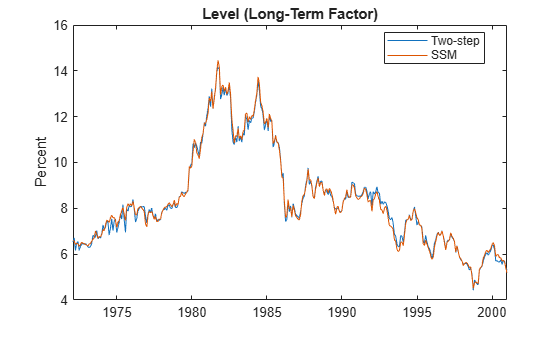plot(dates, [Beta(:,2) EstimatedStates(:,2)]) title('Slope (Short-Term Factor)') ylabel('Percent') legend({'Two-step','SSM'},'Location','best')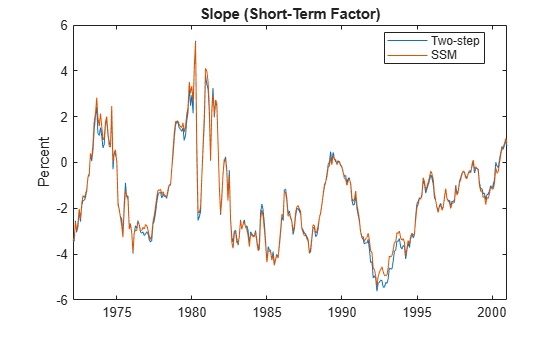plot(dates, [Beta(:,3) EstimatedStates(:,3)]) title('Curvature (Medium-Term Factor)') ylabel('Percent') legend({'Two-step','SSM'},'Location','best')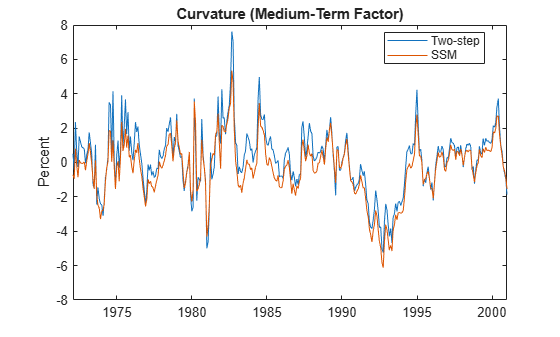The level and slope closely agree. The patterns that the curvature estimates form agree, but the values are slightly off.

Next display the estimated decay rate parameter $\lambda$ associated with the curvature.

lambda % SSM decay rate
lambda = 0.0778 

The estimated decay rate parameter is somewhat larger than the value used by the two-step estimation method, which is 0.0609. $\lambda$ determines the maturity at which the loading on the curvature is maximized. The two-step estimation method fixes $\lambda$ at 0.0609, which reflects the decision to maximize the curvature loading at exactly 2.5 years (30 months). In contrast, the SSM estimates the maximum curvature loading to occur at just less than 2 years (23.1 months).

To see the effects of $\lambda$ on the curvature loading, plot the curvature loading with respect to maturity for each value of $\lambda$.

tau = 0:(1/12):max(maturities); % Maturity (months) decay = [lambda0 lambda]; Loading = zeros(numel(tau), 2); for i = 1:numel(tau) Loading(i,:) = ((1-exp(-decay*tau(i)))./(decay*tau(i))-exp(-decay*tau(i))); end figure plot(tau,Loading) title('Loading on Curvature (Medium-Term Factor)') xlabel('Maturity (Months)') ylabel('Curvature Loading') legend({'\lambda = 0.0609', ['\lambda = ' num2str(lambda)],},'Location','best')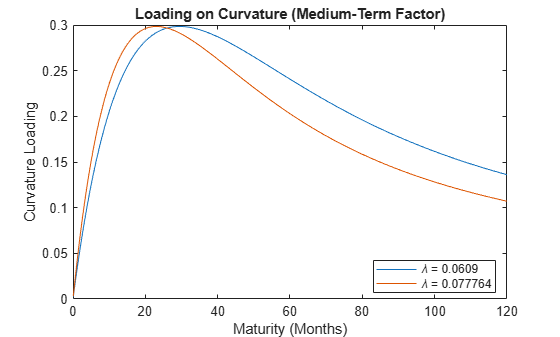The hump-shaped behavior of the curvature loading as a function of maturity reveals why the curvature is interpreted as a medium-term factor. Although differences between the two methods exist, the factors derived from each approach generally agree reasonably closely. Because the one-step SSM/Kalman filter estimation method, in which all model parameters are estimated simultaneously, is more flexible, the method is preferred over the two-step estimation method.

#### Summary of Residuals

Compare the means and standard deviations of the observation equation residuals between the two estimation methods, as in Table 2 of . In the reference, the factor loadings matrix $\Lambda$ is the state measurement sensitivity matrix $C$ of the SSM formulation. Express the results in basis points (bps). This example uses custom functions for display purposes. For details, see Supporting Functions.

ResidualsSSM = Yields - EstimatedStates*EstMdlSSM.C'; Residuals2Step = Yields - Beta*X'; residualMeanSSM = 100*mean(ResidualsSSM)'; residualStdSSM = 100*std(ResidualsSSM)'; residualMean2Step = 100*mean(Residuals2Step)'; residualStd2Step = 100*std(Residuals2Step)'; compareresiduals(maturities,residualMeanSSM, ... residualStdSSM," State-Space Model ",residualMean2Step, ... residualStd2Step," Two-Step ")
 ------------------------------------------------- State-Space Model Two-Step ------------------- ------------------ Standard Standard Maturity Mean Deviation Mean Deviation (Months) (bps) (bps) (bps) (bps) -------- -------- --------- ------- --------- 3.0000 -12.6440 22.3639 -7.3922 14.1709 6.0000 -1.3392 5.0715 2.1914 7.2895 9.0000 0.4922 8.1084 2.7173 11.4923 12.0000 1.3059 9.8672 2.5472 11.1200 15.0000 3.7130 8.7073 4.2189 9.0558 18.0000 3.5893 7.2946 3.5515 7.6721 21.0000 3.2308 6.5112 2.7968 7.2221 24.0000 -1.3996 6.3890 -2.1168 7.0764 30.0000 -2.6479 6.0614 -3.6923 7.0129 36.0000 -3.2411 6.5915 -4.4095 7.2674 48.0000 -1.8508 9.7019 -2.9761 10.6242 60.0000 -3.2857 8.0349 -4.2314 9.0296 72.0000 1.9737 9.1370 1.2238 10.3745 84.0000 0.6935 10.3689 0.1196 9.8012 96.0000 3.4873 9.0440 3.0626 9.1220 108.0000 4.1940 13.6422 3.8936 11.7942 120.0000 -1.3074 16.4545 -1.5043 13.3544 

Because the SSM yields lower residual means and standard deviations for most maturities, the SSM provides a better fit than the two-step estimation method, particularly for maturities 6 to 60 months.

### Forecast Estimated SSM

To forecast the estimated SSM EstMdlSSM, you can implement minimum mean square error (MMSE) forecasting or Monte Carlo simulation methods. The SSM functionality supports both methods.

Because the Diebold-Li model depends only on the estimated factors, you forecast the yield curve by forecasting each factor. Also, because the estimated SSM is based on offset yields, you must compensate for the offset adjustment when forecasting or simulating the model, as described in Inferred Factors.

#### Deterministic MMSE Forecasts

Compute MMSE forecasts by performing the following actions:

1. Pass EstMdlSSM and the deflated yields $\stackrel{\sim }{\text{\hspace{0.17em}}{\mathit{y}}_{\mathit{t}}}$ to the forecast function. forecast returns the MMSE forecasts of the deflated yields 1,2,...,12 months into the future.

2. Compute the forecasted yields by adding the estimated offset $C\mu$ to the deflated, forecasted yields.

fh = 12; % Forecast horizon (months) [ForecastedDeflatedYields,FMSE] = forecast(EstMdlSSM,fh,DeflatedYields); MMSEForecasts = ForecastedDeflatedYields + intercept';

The forecasted yield curves MMSEForecasts is a 12-by-17 matrix; each row corresponds to a period in the forecast horizon and each column corresponds to a maturity.

#### Monte Carlo Forecasts

An advantage of Monte Carlo forecast over MMSE forecasts is that you can use the large sample obtained by the Monte Carlo method to study characteristics of the forecast distribution.

To forecast the yield curves by performing Monte Carlo simulation, follow this general procedure:

1. Obtain factor estimates and their covariance matrix at period $\mathit{T}$, which is the end of the in-sample data and the last period before the forecast horizon starts, to initialize the Monte Carlo simulation. Such values ensure the simulation begins with the latest available information. The estimates and covariance matrix correspond to the deflated yields. Specify the initial values in the estimated ssm model object.

2. For all maturities, draw many sample paths of deflated yields into the forecast horizon.

3. Inflate the simulated, deflated yields.

4. For each period in the forecast horizon and maturity, compute summary statistics of the inflated yields over the simulated paths.

Obtain period $\mathit{T}$ factor estimates and their covariance matrix by passing estimated SSM EstMdlSSM and the deflated yields $\stackrel{\sim }{\text{\hspace{0.17em}}{\mathit{y}}_{\mathit{t}}}$ to smooth and return the output structure array containing all estimates and covariances by period. Extract the final element of the fields corresponding to the smoothed estimates.

[~,~,results] = smooth(EstMdlSSM,DeflatedYields); Mean0 = results(end).SmoothedStates; Cov0 = results(end).SmoothedStatesCov; Cov0 = (Cov0 + Cov0')/2; % Ensure covariance is symmetric

results is a $\mathit{T}$-by-1 structure array containing various smoothed estimates and inferences. Because the extracted state mean and covariance occur at the end of the historical data set, you can alternatively use the filter function, instead of smooth, to obtain equivalent initial state values from which to forecast.

Set the initial state mean Mean0 and covariance Cov0 properties of the SSM EstMdlSSM to the appropriate smoothed estimates.

EstMdlSSM.Mean0 = Mean0; % Initial state mean EstMdlSSM.Cov0 = Cov0; % Initial state covariance

Draw 100,000 sample paths of deflated yields from the estimated SSM into the forecast horizon. Inflate the simulated, deflated yields.

rng('default') % For reproducibility numPaths = 100000; SimulatedDeflatedYields = simulate(EstMdlSSM,fh,numPaths); SimulatedYields = SimulatedDeflatedYields + intercept';

SimulatedYields is a 12-by-17-by-100,000 numeric array:

• Each row is a period in the forecast horizon.

• Each column is a maturity.

• Each page is a random draw from the forecast distribution. In other words, each page represents the future evolution of a simulated yield curve over a 12-month forecast horizon.

Compute the sample means and standard deviations over the 100,000 draws.

MCForecasts = mean(SimulatedYields,3); MCStandardErrors = std(SimulatedYields,[],3);

MCForecasts and MCStandardErrors are the Monte Carlo simulation analogs of the MMSE forecasts MMSEForecasts and standard errors FMSE, respectively.

Visually compare the MMSE and Monte Carlo simulation forecasts and corresponding standard errors.

figure plot(maturities,[MCForecasts(fh,:)' MMSEForecasts(fh,:)']) title('12-Month-Ahead Forecasts: Monte Carlo vs. MMSE') xlabel('Maturity (Months)') ylabel('Percent') legend({'Monte Carlo','MMSE'},'Location','best')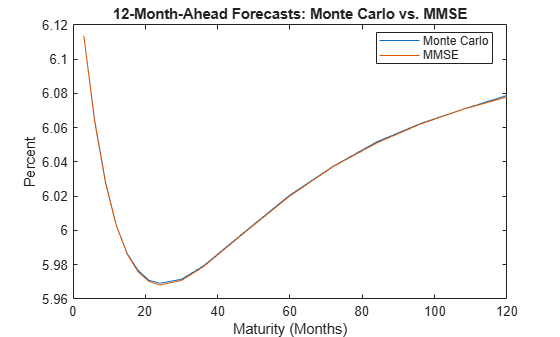figure plot(maturities,[MCStandardErrors(fh,:)' sqrt(FMSE(fh,:))']) title('12-Month-Ahead Forecast Standard Errors: Monte Carlo vs. MMSE') xlabel('Maturity (Months)') ylabel('Percent') legend({'Monte Carlo','MMSE'},'Location','best')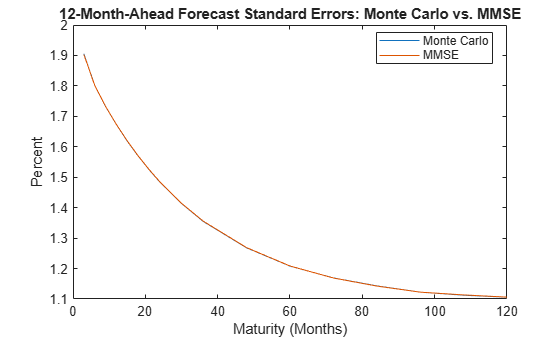The estimates are effectively identical.

A benefit of Monte Carlo simulation is that it enables an analysis of the distribution of the yields beyond their mean and standard error. Monte Carlo simulation provides additional insight into how the distribution affects the distribution of other variables dependent on it. For example, the insurance industry commonly uses the simulation of yield curves to assess the distribution of profits and losses associated with annuities and pension contracts.

Display the distribution of the simulated 12-month yield at 1, 6, and 12 months into the future, similar to the forecasting experiment in Tables 4 through 6 of .

index12 = find(maturities == 12); % Page index of 12-month yield bins = 0:0.2:12; figure subplot(3,1,1) histogram(SimulatedYields(1,index12,:),bins,'Normalization','pdf') title('PDF of 12-Month Yield') xlabel('Yield 1 Month into the Future (%)') subplot(3,1,2) histogram(SimulatedYields(6,index12,:),bins,'Normalization','pdf') xlabel('Yield 6 Months into the Future (%)') ylabel('Probability') subplot(3,1,3) histogram(SimulatedYields(12,index12,:),bins,'Normalization','pdf') xlabel('Yield 12 Months into the Future (%)')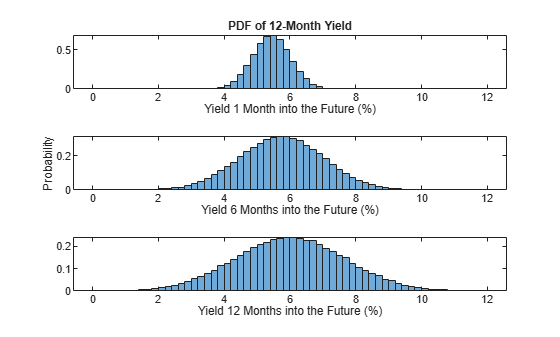Forecasts further out in the forecast horizon are more uncertain than forecasts closer to the end of the in-sample period.

### Augment Yield Curve Model with Macro Factors

The yields-macro model extends the yields-only model by including macroeconomic and financial factors. A minimum set of variables that characterize the economic activities include manufacturing capacity utilization (CU, ${\mathit{z}}_{1,\mathit{t}}$) , the federal funds rate (FEDFUNDS, ${\mathit{z}}_{2,\mathit{t}}$) , and annual price inflation (PI, ${\mathit{z}}_{3,\mathit{t}}$) , which interact with the level, slope, and curvature factors in a vector autoregression. The augmented SSM is

$\begin{array}{c}\left({f}_{t}-\mu \right)=A\left({f}_{t-1}-\mu \right)+{\eta }_{t}\\ \left[\begin{array}{c}{y}_{t}\\ {z}_{t}\end{array}\right]=\left[\begin{array}{cc}\Lambda & 0\\ 0& I\end{array}\right]\phantom{\rule{0.2777777777777778em}{0ex}}{f}_{t}+\left[\begin{array}{c}{w}_{t}\\ 0\end{array}\right]\end{array},$

where ${f}_{t}=\left[{L}_{t},{S}_{t},{C}_{t},{z}_{1,t},{z}_{2,t},{z}_{3,t}{\right]}^{\prime }$ and ${\mathit{z}}_{\mathit{t}}={\left[{z}_{1,t},{z}_{2,\mathit{t}},{z}_{3,\mathit{t}}\right]}^{\prime }$. The dimensions of $A$ and $\mu$ increase to 6-by-6 and 6-by-1, respectively. The observation equation ${\mathit{y}}_{\mathit{t}}=\left[\begin{array}{cc}\Lambda & 0\end{array}\right]{\mathit{f}}_{\mathit{t}}+{\mathit{w}}_{\mathit{t}}$ indicates that the level, slope, and curvature factors sufficiently distill the information in the yield curve. Also, ${z}_{t}=\left(\begin{array}{c}0\phantom{\rule{0.5em}{0ex}}I\end{array}\right){f}_{t}$ indicates that the macroeconomic variables are observed without measurement error. The SSM framework accounts for missing observations, denoted by NaN values in the data, by substituting estimates derived from the Kalman filter. Like the yields-only formulation, the white noise processes ${\eta }_{t}$ and ${w}_{t}$ have the distribution

$\left[\begin{array}{c}{\eta }_{t}\\ {w}_{t}\end{array}\right]\phantom{\rule{0.5em}{0ex}}\sim WN\left(\left[\begin{array}{c}0\\ 0\end{array}\right],\left[\begin{array}{cc}Q& 0\\ 0& H\end{array}\right]\right),$

where $Q$ is a 6-by-6 symmetric positive definite matrix, and $H$ is diagonal.

The formulation supported by the SSM functionality is

$\begin{array}{l}{x}_{t}={A}_{t}{x}_{t-1}+{B}_{t}{u}_{t}\\ {\zeta }_{t}={C}_{t}{x}_{t}+{D}_{t}{\epsilon }_{t},\end{array}$

where the states are the mean-adjusted factors, namely ${x}_{t}={f}_{t}-\mu$. The deflated observations are

${\zeta }_{t}=\left[\begin{array}{c}{y}_{t}\\ {z}_{t}\end{array}\right]-\left[\begin{array}{cc}\Lambda & 0\\ 0& I\end{array}\right]\phantom{\rule{0.2777777777777778em}{0ex}}\mu .$

The model contains 81 unknown parameters:

• $A$ contains 36 parameters.

• $Q$ contains 21 parameters.

• $H$ contains 17 parameters.

• $\mu$ contains 6 parameters.

• $\Lambda$ contains the scalar $\lambda$.

The mapping function Example_YieldsMacro.m maps a parameter vector to SSM parameter matrices, deflates the observations to account for the means of each factor, and imposes constraints on the covariance matrices. For more details, open Example_YieldsMacro.m.

### Estimate Yields-Macro Diebold-Li SSM

The yields data set Data_DieboldLi.mat additionally contains observations of the macroeconomic series, which are available in the Federal Reserve Economic Database (FRED) . The macroeconomic series in Data_DieboldLi.mat are not identical to the corresponding series in , but this example reproduces most of those empirical results.

Extract the macroeconomic series from the data, and determine variable dimensions.

Macro = [DataTimeTable.CU DataTimeTable.FEDFUNDS DataTimeTable.PI]; numBonds = size(Yields,2); numMacro = size(Macro,2); numStates = 3 + numMacro;

The estimation of 81 parameters by maximum likelihood is computationally challenging, but it is possible with carefully specified initial values for numerical optimization.

To obtain reasonable initial values, fit a vector autoregression model (the state equation) to the estimated factors Beta and the macroeconomic series Macro. Then, extract and process the estimates from the estimated model.

MdlVAR0 = varm(numStates,1); EstMdlVAR0 = estimate(MdlVAR0,[Beta Macro]); A0 = EstMdlVAR0.AR{1}; B0 = chol(EstMdlVAR0.Covariance,'lower'); D0 = std(Residuals); mu0 = [mean(Beta) mean(Macro,'omitnan')]; p0Macro = [A0(:); nonzeros(B0); D0(:); mu0(:); lambda0];

The estimate function estimates unknown parameters of an SSM by optimizing the loglikelihood using fminunc or fmincon. At each iteration, estimate computes the loglikelihood by applying the Kalman filter. This complex optimization task can require numbers of iterations and function evaluations that are larger than their default. Also, the dimension of the observation vector is substantially larger than the state vector, and therefore the method that treats a multivariate series as univariate can improve run-time performance.

Create an optimization options object that specifies a maximum of 1000 iterations and 50,000 function evaluations for unconstrained optimization. Create an SSM by passing, to ssm, the parameter-to-matrix mapping function Example_YieldsMacro as an anonymous function with an input argument representing the parameter vector params. The additional input arguments of the mapping function specify the yields, maturity, and macroeconomic series information statically. The inputs initialize the model for estimation.

options = optimoptions('fminunc','MaxIterations',1000,'MaxFunctionEvaluations',50000); MdlMacro = ssm(@(params)Example_YieldsMacro(params,Yields,Macro,maturities));

Estimate the yields-macro Diebold-Li SSM. Turn off the estimation display and specify the optimization options and the method that treats multivariate series as univariate.

[EstMdlMacro,estParamsMacro,EstParamCovMacro,logLMacro] = estimate(MdlMacro,[Yields Macro],p0Macro, ... 'Display','off','Options',options,'Univariate',true);

Because the SSM operates on the mean-adjusted factors, you must deflate (intercept-adjust) the observations before operating on the estimated model and the observations. Get the estimated SSM coefficients and the deflated data by specifying the estimated parameters as inputs to Example_YieldsMacro.m. Display the estimated state-transition matrix $\mathit{A}$ and state-disturbance covariance matrix $\mathit{B}{\mathit{B}}^{\prime }$, which account for the macroeconomic factors.

[A,B,C,~,~,~,~,deflatedData] = Example_YieldsMacro(estParamsMacro,Yields,Macro,maturities); A
A = 6×6 0.8986 -0.0624 -0.0245 -0.0061 0.0756 0.0134 -0.4325 0.4805 0.0314 0.0312 0.3768 0.0260 0.1355 0.1182 0.8455 0.0111 -0.0918 0.0023 0.0679 -0.0257 0.0023 0.9982 -0.0655 -0.0202 -0.0064 -0.0887 0.0106 0.0461 0.9958 0.0456 -0.0348 -0.0314 -0.0150 0.0268 0.0364 0.9940 
B*B'
ans = 6×6 0.0914 -0.0226 0.0466 0.0370 0.0327 0.0114 -0.0226 0.3041 0.0076 0.0772 0.2241 -0.0051 0.0466 0.0076 0.8105 0.0259 0.1690 0.0023 0.0370 0.0772 0.0259 0.3710 0.1425 0.0254 0.0327 0.2241 0.1690 0.1425 0.4494 0.0128 0.0114 -0.0051 0.0023 0.0254 0.0128 0.0475 

Given the full-sample data and a fitted SSM, the Kalman smoother smooth provides state estimates. The smooth function enables you to evaluate the measurement errors of the yields. For each maturity, obtain measurement error estimates by computing the mean and standard deviation of the residuals. Visually compare the measurement error estimates of the estimated yields-only and yields-macro models.

StatesMacro = smooth(EstMdlMacro,deflatedData); ResidualsMacro = deflatedData - StatesMacro*C'; residualMeanMacro = 100*mean(ResidualsMacro,'omitnan')'; residualStdMacro = 100*std(ResidualsMacro,'omitnan')'; compareresiduals(maturities,residualMeanSSM,residualStdSSM, ... " Yields-Only Model ",residualMeanMacro(1:numBonds), ... residualStdMacro(1:numBonds),"Yields-Macro Model")
 ------------------------------------------------- Yields-Only Model Yields-Macro Model ------------------- ------------------ Standard Standard Maturity Mean Deviation Mean Deviation (Months) (bps) (bps) (bps) (bps) -------- -------- --------- ------- --------- 3.0000 -12.6440 22.3639 -12.5379 22.2240 6.0000 -1.3392 5.0715 -1.2658 4.8526 9.0000 0.4922 8.1084 0.5387 8.1444 12.0000 1.3059 9.8672 1.3310 9.8812 15.0000 3.7130 8.7073 3.7212 8.7603 18.0000 3.5893 7.2946 3.5846 7.3145 21.0000 3.2308 6.5112 3.2168 6.4719 24.0000 -1.3996 6.3890 -1.4201 6.3520 30.0000 -2.6479 6.0614 -2.6745 6.0864 36.0000 -3.2411 6.5915 -3.2675 6.6115 48.0000 -1.8508 9.7019 -1.8663 9.7266 60.0000 -3.2857 8.0349 -3.2855 8.0124 72.0000 1.9737 9.1370 1.9896 9.1110 84.0000 0.6935 10.3689 0.7231 10.3780 96.0000 3.4873 9.0440 3.5285 9.1650 108.0000 4.1940 13.6422 4.2447 13.7447 120.0000 -1.3074 16.4545 -1.2488 16.5814 

The yields-only model fits the yield curve data well, but the yields-macro model performs better.

Because the macroeconomic variables are perfectly observed, ensure that their estimated measurement errors are close to zero, to within machine precision.

residualMeanMacro(end-numMacro+1:end)'
ans = 1×3 10-14 × -0.0632 0.5795 0.3373 

### Characterize Yields-to-Macro and Macro-to-Yields Links

#### Impulse Response Functions of Yields-Macro Model

For an SSM, the impulse response function (IRF) measures the dynamic effects on the state and measurement equations due to an unanticipated shock to each state disturbance. In the transition equation ${x}_{t}=A{x}_{t-1}+B{u}_{t}$, the IRF is the partial derivative of ${\mathit{x}}_{\mathit{t}}$, $\mathit{t}=1,2,...$ with respect to ${u}_{1}$. The SSM IRF functionality, irf and irfplot, performs the following actions:

• Apply the state shock during period 1.

• Normalize the shock variance to one; The state-disturbance-loading matrix $B$ determines any contemporaneous effects.

• Return responses for periods $\mathit{t}=1,2,...$.

For the yields-macro model, the matrix $B$ is identified up to $Q=B{B}^{\prime }$. The IRF of the yields-macro SSM requires identification conditions, such as a recursive ordering of state variables ${L}_{t},{S}_{t},{C}_{t},{z}_{1,t},{z}_{2,t},{z}_{3,t}$ so that $B$ is a lower triangular matrix. The rationale is that yields are dated at the beginning of each month, while macroeconomic data is published with a time lag. Consequently, the yields have a contemporaneous effect on the macroeconomic variables, but not vice versa.

Use irfplot to plot the IRF of the following groups of variables:

• Yield-curve responses to yield-curve shocks

• Macro responses to macro shocks

• Macro responses to yield-curve shocks

• Yield-curve responses to macro shocks

For each plot, specify 90% confidence bounds and plot responses for the first 30 periods.

figure irfplot(MdlMacro,'Params',estParamsMacro,'EstParamCov',EstParamCovMacro, ... 'PlotU',1:3,'PlotX',1:3,'PlotY',[],'NumPeriods',30,'Confidence',0.9); sgtitle('Yield-Curve Responses to Yield-Curve Shocks')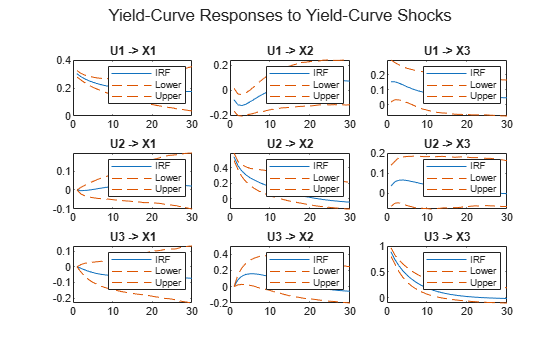figure irfplot(MdlMacro,'Params',estParamsMacro,'EstParamCov',EstParamCovMacro, ... 'PlotU',4:6,'PlotX',4:6,'PlotY',[],'NumPeriods',30,'Confidence',0.9); sgtitle('Macro Responses to Macro Shocks')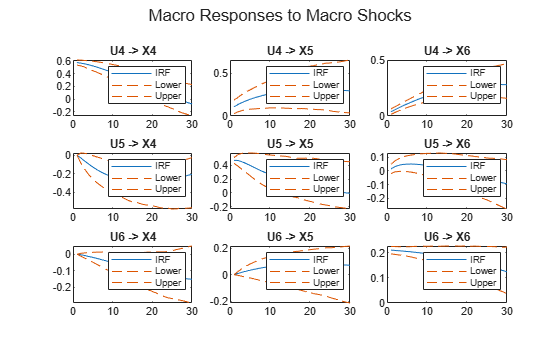figure irfplot(MdlMacro,'Params',estParamsMacro,'EstParamCov',EstParamCovMacro, ... 'PlotU',1:3,'PlotX',4:6,'PlotY',[],'NumPeriods',30,'Confidence',0.9); sgtitle('Macro Responses to Yield-Curve Shocks')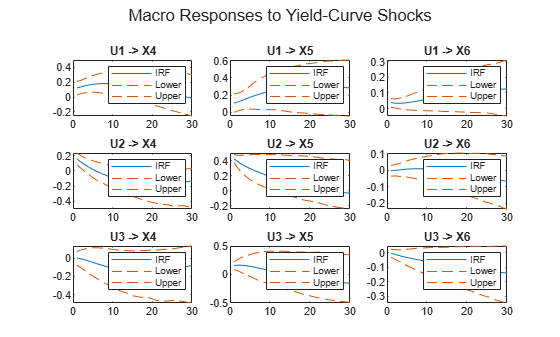figure irfplot(MdlMacro,'Params',estParamsMacro,'EstParamCov',EstParamCovMacro, ... 'PlotU',4:6,'PlotX',1:3,'PlotY',[],'NumPeriods',30,'Confidence',0.9); sgtitle('Yield-Curve Responses to Macro Shocks')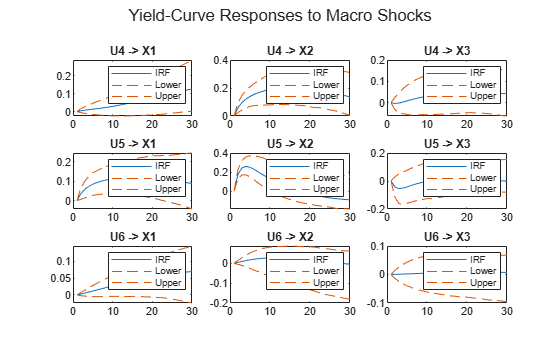#### Variance Decompositions of Yields-Macro Model

The forecast error variance decomposition (FEVD) provides information about the relative importance of each shock in affecting the forecast error variance of a response variable. Regarding the macro and yield curve interactions, FEVD analyzes whether the macro factors are influential, compared to the idiosyncratic variation in the yield curve. In the SSM, the disturbances in the transition and measurement equations cause the forecast variance of the observations. In the presence of a nonzero, observation-innovation coefficient matrix $D$, the decomposition does not sum to one because the remaining portion is attributable to the observation noise covariance $D{D}^{\prime }$. To force the sum to one, you can account for the observation noise by rescaling the FEVD.

Compute FEVD of the 12-month yield at the forecast horizons of 1, 12, and 60 months by using the fevd function. Because fevd applies the unit shock at period 1, the forecast horizon starts at period 2. Normalize each FEVD to sum to one.

Decomposition = fevd(EstMdlMacro,'NumPeriods',61); idxyield12 = find(maturities == 12); d1 = Decomposition(1+1,:,idxyield12); d12 = Decomposition(1+12,:,idxyield12); d60 = Decomposition(1+60,:,idxyield12); d1 = d1./sum(d1); d12 = d12./sum(d12); d60 = d60./sum(d60); displayfevd([d1; d12; d60],"12-Month Yield")
Variance Decomposition, 12-Month Yield -------------------------------- Horizon L S C CU FEDFUNDS PI 1.0000 0.2977 0.4397 0.2189 0.0037 0.0400 0.0001 12.0000 0.3080 0.2302 0.1345 0.0986 0.2211 0.0076 60.0000 0.3874 0.0855 0.1258 0.2836 0.0932 0.0245 

The FEVD results indicate that less than 5 percent of the variation is attributable to the macroeconomic factors at the 1-month horizon. However, the macroeconomic factors are more influential at longer horizons. At the 12-month and 60-month horizons, the macroeconomic factors account for about 30 and 40 percent of the variation, respectively.

Compute FEVD of the manufacturing capacity utilization series at the forecast horizons of 1, 12, and 60 months. Normalize each FEVD to sum to one.

cu1 = Decomposition(1+1,:,numBonds+1); cu12 = Decomposition(1+12,:,numBonds+1); cu60 = Decomposition(1+60,:,numBonds+1); displayfevd([cu1; cu12; cu60],"Capacity Utilization")
Variance Decomposition, Capacity Utilization -------------------------------- Horizon L S C CU FEDFUNDS PI 1.0000 0.0466 0.0531 0.0001 0.8989 0.0013 0.0000 12.0000 0.0913 0.0260 0.0224 0.7465 0.1084 0.0054 60.0000 0.1015 0.0605 0.0595 0.5149 0.1786 0.0849 

In contrast to the FEVD of the 12-month yield, the variance decompositions of manufacturing capacity utilization show that the level, slope, and curvature factors account for a small portion of the variation at each period in the forecast horizon. The variance decompositions of the other two macroeconomic variables exhibit the same pattern.

### Augment Diebold-Li SSM with Exogenous Macroeconomic Variables

In the fitted yields-macro model, the estimated parameters in the bottom-left corner of the transition matrix $A\left(4:6,1:3\right)$ are not individually significant. This result motivates a model with a more parsimonious specification, specifically with the constraint $A\left(4:6,1:3\right)=0$ and a diagonal covariance matrix $Q$. In the constrained model, the macroeconomic variables are exogenous, which means that the macroeconomic factors impact the yield curve factors, but the link is unilateral because the yields-to-macro link is absent.

The mapping function Example_YieldsExogenous.m maps a parameter vector to SSM parameter matrices, deflates the observations to account for the means of each factor, imposes constraints on the covariance matrices, and includes exogenous variables in the state equation. For more details, open Example_YieldsExogenous.m.

Create a Diebold-Li SSM augmented with exogenous macroeconomic variables by passing, to ssm, the parameter-to-matrix mapping function Example_YieldsExogenous as an anonymous function with an input argument representing the parameter vector params. The additional input arguments of the mapping function specify the yields, maturity, and macroeconomic series information statically, which initialize the model for estimation.

MdlExogenous = ssm(@(params)Example_YieldsExogenous(params,Yields,Macro,maturities));

The constrained MdlExogenous model has 57 unknown parameters.

Estimate the Diebold-Li SSM augmented with exogenous variables. Turn off the estimation display and specify the same options and initial values as specified for the estimation of the yields-macro SSM.

Mask = true(numStates); Mask(4:end,1:3) = false; param0Exogenous = [A0(Mask); diag(B0); D0(:); mu0(:); lambda0]; [EstMdlExogenous,estParamsExogenous,~,logLExogenous] = estimate(MdlExogenous,[Yields Macro], ... param0Exogenous,'Display','off','Options',options,'Univariate',true); logLMacro
logLMacro = 2.7401e+03 
logLExogenous
logLExogenous = 2.5312e+03 

The maximized loglikelihood of EstMdlExogenous is necessarily lower than that of the full model EstMdlMacro, which has 81 parameters.

Get the estimated SSM coefficients and the deflated data by specifying the estimated parameters as inputs to Example_YieldsExogenous.m. Display the estimated state-transition matrix $\mathit{A}$.

[AExogenous,~,CExogenous,~,~,~,~,deflatedDataExogenous] = Example_YieldsExogenous(estParamsExogenous, ... Yields,Macro,maturities); AExogenous
AExogenous = 6×6 0.8963 -0.0679 -0.0203 -0.0050 0.0776 0.0149 -0.4426 0.4733 0.0315 0.0301 0.3836 0.0263 0.1406 0.1286 0.8351 0.0073 -0.0955 -0.0024 0 0 0 0.9785 -0.0447 -0.0254 0 0 0 0.0276 0.9600 0.0370 0 0 0 0.0289 0.0050 0.9961 

For each maturity, obtain measurement error estimates by computing the mean and standard deviation of the residuals. Visually compare the measurement error estimates of the estimated yields-macro model and the Diebold-Li model augmented with exogenous variables.

StatesExogenous = smooth(EstMdlExogenous,deflatedDataExogenous); ResidualsExogenous = deflatedDataExogenous - StatesExogenous*CExogenous'; residualMeanExogenous = 100*mean(ResidualsExogenous,'omitnan')'; residualStdExogenous = 100*std(ResidualsExogenous,'omitnan')'; compareresiduals(maturities,residualMeanMacro(1:numBonds),residualStdMacro(1:numBonds), ... "Yields-Macro Model",residualMeanExogenous(1:numBonds),... residualStdExogenous(1:numBonds)," Exogenous Model")
 ------------------------------------------------- Yields-Macro Model Exogenous Model ------------------- ------------------ Standard Standard Maturity Mean Deviation Mean Deviation (Months) (bps) (bps) (bps) (bps) -------- -------- --------- ------- --------- 3.0000 -12.5379 22.2240 -12.5684 22.3726 6.0000 -1.2658 4.8526 -1.2984 4.7886 9.0000 0.5387 8.1444 0.5108 8.0531 12.0000 1.3310 9.8812 1.3111 9.9134 15.0000 3.7212 8.7603 3.7106 8.7239 18.0000 3.5846 7.3145 3.5829 7.2634 21.0000 3.2168 6.4719 3.2229 6.4634 24.0000 -1.4201 6.3520 -1.4078 6.3814 30.0000 -2.6745 6.0864 -2.6548 6.0777 36.0000 -3.2675 6.6115 -3.2467 6.5898 48.0000 -1.8663 9.7266 -1.8568 9.7271 60.0000 -3.2855 8.0124 -3.2958 8.0565 72.0000 1.9896 9.1110 1.9578 9.1354 84.0000 0.7231 10.3780 0.6715 10.3317 96.0000 3.5285 9.1650 3.4597 8.9413 108.0000 4.2447 13.7447 4.1615 13.6561 120.0000 -1.2488 16.5814 -1.3441 16.4350 

The estimated transition matrices of the models are similar. EstMdlExogenous results in larger measurement errors of yields at some maturities, as compared to the measurement errors of EstMdlMacro. Despite this result, EstMdlExogenous fits the curve well overall.

In summary, the yields-macro and Diebold-Li model augmented with exogenous variables have significant statistical and economic differences. Despite the large difference in the fitted loglikelihoods of the models, the fitted yield curve of the SSM augmented with exogenous variables is not necessarily inferior to the yield curve of the yields-macro SSM.

### Supporting Functions

Local functions facilitate several command-line displays for this example.

function compareresiduals(maturities,residualMeanL,residualStdL,tL,residualMeanR,residualStdR,tR) header = [" -------------------------------------------------"; ... " " + tL + " " + tR;... " ------------------- ------------------"; ... " Standard Standard"; ... " Maturity Mean Deviation Mean Deviation"; ... " (Months) (bps) (bps) (bps) (bps) "; ... " -------- -------- --------- ------- ---------"]; tab = [maturities residualMeanL residualStdL residualMeanR residualStdR]; fprintf("%s\n",header) disp(tab) end function displayfevd(d,t) header = ["Variance Decomposition, " + t; ... "--------------------------------"; ... " Horizon L S C CU FEDFUNDS PI"]; tab = [[1;12;60] d]; fprintf("%s\n",header) disp(tab) end

 Diebold, F.X., and C. Li. "Forecasting the Term Structure of Government Bond Yields." Journal of Econometrics. Vol. 130, No. 2, 2006, pp. 337–364.

 Diebold, F. X., G. D. Rudebusch, and B. Aruoba (2006), "The Macroeconomy and the Yield Curve: A Dynamic Latent Factor Approach." Journal of Econometrics. Vol. 131, 2006, pp. 309–338.

 Nelson, R. C., and A. F. Siegel. "Parsimonious Modeling of Yield Curves." Journal of Business. Vol. 60, No. 4, 1987, pp. 473–489.

 U.S. Federal Reserve Economic Data (FRED), Federal Reserve Bank of St. Louis, https://fred.stlouisfed.org/.

 Board of Governors of the Federal Reserve System (US), Effective Federal Funds Rate [FEDFUNDS], retrieved from FRED, Federal Reserve Bank of St. Louis; https://fred.stlouisfed.org/series/FEDFUNDS, March 1, 2021.

 U.S. Bureau of Economic Analysis, Personal Consumption Expenditures: Chain-type Price Index [PCEPI], retrieved from FRED, Federal Reserve Bank of St. Louis; https://fred.stlouisfed.org/series/PCEPI, March 1, 2021.

 Board of Governors of the Federal Reserve System (US), Capacity Utilization: Manufacturing (SIC) [CUMFNS], retrieved from FRED, Federal Reserve Bank of St. Louis; https://fred.stlouisfed.org/series/CUMFNS, March 1, 2021.